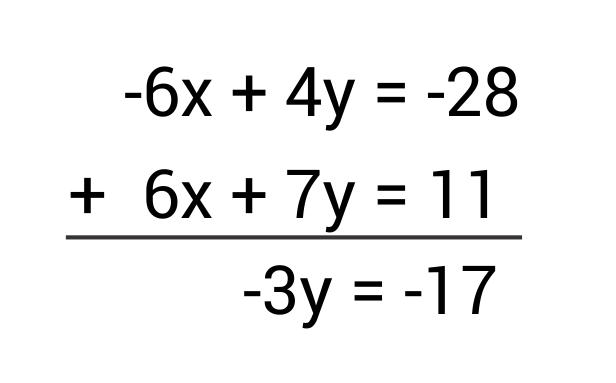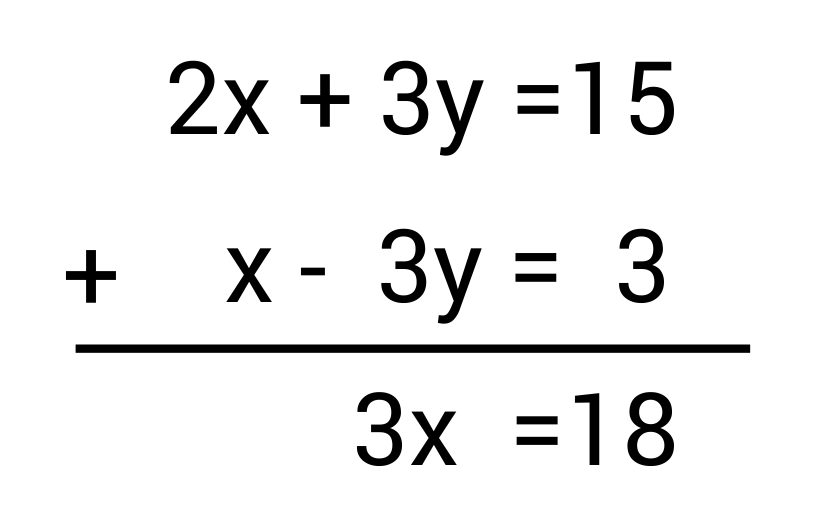# Top 3 Methods for Solving Systems of EquationsHey, guys! Welcome to this video over comparing different methods for solving a system of equations.

If you recall, a system of equations is when you have more than one equation with unknown variables in a given problem. So, in order to solve that problem, you need to be able to find the value of all the variables in each equation.

There are three different ways that you could do this: the substitution method, elimination method, and using an augmented matrix.

In this video, I’m assuming that you already know how to perform each method, so I want to spend a lot of time explaining not how to do them but rather when to use each method.

First, I will verbally tell you when to use each method, then I will write out three different examples, and we will decide together which method is most efficient for each system.

### When to Use the Substitution Method

You should use the substitution method when one of the variables in one of your equations has already been isolated (it has a coefficient of 1).

### When to Use the Elimination Method

You should use the elimination method when the same variables in all of the equations share the same coefficient, or when they share the same but negative coefficient.

### When to Use an Augmented Matrix

You would use an augmented matrix when the substitution and elimination method are either impractical or impossible altogether.

Now, let’s look at three different systems, and use what we’ve just learned to think through which method is most useful for each system.

EXAMPLE SYSTEM #1
$$5x – 58y = -883$$
$$-5x + 2y = -13$$

EXAMPLE SYSTEM #2
$$9x + 4y = 65$$
$$x – 18y = -2$$

EXAMPLE SYSTEM #3
$$2x + 7y – 3z = 47$$
$$x – 4y + 8z = -33$$
$$7x + 2y +10z = 11$$

So, what we will do is go through each system, decide which method would be most efficient, and then solve with that method.

## Elimination Method Example

Alright, let’s look at this first equation.

$$5x – 58y = -883$$
$$-5x + 2y = -13$$

Now, thinking back to the explanation I gave on when to use each method, notice what I said about elimination: “You should use the elimination method when the same variables in all of the equations share the same coefficient, or when they share the same but negative coefficient.”

Well this exact thing is true in the case of this particular system. So, let’s solve this system using elimination.

$$-56y = -896$$
$$y = 16$$

Now, we plug our y variable back into one of the original equations. I’ll plug it into the first.

$$5x – 58(16) = -883$$
$$5x – 928 = -883$$
$$5x = 45$$
$$x = 9$$

Great, so we’ve solved this system using elimination, because our same two variables had the same coefficient or when they share the same but negative coefficient (like in our case).

## Substitution Method Example

Let’s move on to system #2.

$$9x + 4y = 65$$
$$x – 18y = -2$$

Alright, so again, let’s think back on what was said in our explanation on when to use each method. Recall what was said about substitution: “You should use the substitution method when one of the variables in one of your equations has already been isolated.”

Well, such is the case with this system. Our $$x$$-variable in our second equation has a coefficient of 1. So, let’s solve this system using substitution.

$$9(18y – 2) + 4y = 65$$
$$162y – 18 + 4y = 65$$
$$166y = 83$$
$$y = \frac{1}{2}$$
$$x = 18(\frac{1}{2}) – 2$$
$$x = 7$$

That was very simple to solve using substitution. Remember, the signifier to help you know when to use it is if one of the equations has a variable that is already isolated.

## Augmented Matrix Example

Let’s look at our last system, system #3.

$$2x + 7y – 3z = 47$$
$$x – 4y + 8z = -33$$
$$7x + 2y +10z = 11$$

Remember, what we said about when to use an augmented matrix. Well, right now is a good time. Using elimination or substitution for that matter would take a lot more work than would using an augmented matrix.

So, let’s set up our matrix and solve.

$$\begin{bmatrix} \left.\begin{matrix} 2& 7& -3\\ 1& -4& 8\\ 7& 2& 10 \end{matrix}\right| & \begin{matrix} 47\\ -33\\ 11 \end{matrix} \end{bmatrix}$$
$$R_{1}\leftrightarrow R_{2}$$
$$\begin{bmatrix} \left.\begin{matrix} 1& -4& 8\\ 2& 7& -3\\ 7& 2& 10 \end{matrix}\right| & \begin{matrix} -33\\ 47\\ 11 \end{matrix} \end{bmatrix}$$
$$\begin{matrix} \\ -2R_{1}+R_{2}=R_{2}\\ -7R_{1}+R_{3}=R_{3} \end{matrix}\begin{bmatrix} \left.\begin{matrix} 1& -4& 8\\ 0& 15& -19\\ 0& 30& -46 \end{matrix}\right| & \begin{matrix} -33\\ 113\\ 242 \end{matrix} \end{bmatrix}$$
$$\begin{matrix} \\ \frac{R_{2}}{15}=R_{2}\\ \end{matrix}\begin{bmatrix} \left.\begin{matrix} 1& -4& 8\\ 0& 1& \frac{-19}{15}\\ 0& 30& -46 \end{matrix}\right| & \begin{matrix} -33\\ \frac{113}{15}\\ 242 \end{matrix} \end{bmatrix}$$
$$\begin{matrix} 4R_{2}+R_{1}=R_{1}\\ \\ -30R_{2}+R_{3}=R_{3} \end{matrix}\begin{bmatrix} \left.\begin{matrix} 1& 0& \frac{44}{15}\\ 0& 1& \frac{-19}{15}\\ 0& 0& -8 \end{matrix}\right| & \begin{matrix} \frac{-43}{15}\\ \frac{113}{15}\\ 16 \end{matrix} \end{bmatrix}$$
$$\begin{matrix} \\ \\ \frac{R_{3}}{-8}=R_{3} \end{matrix}\begin{bmatrix} \left.\begin{matrix} 1& 0& \frac{44}{15}\\ 0& 1& \frac{-19}{15}\\ 0& 0& 1 \end{matrix}\right| & \begin{matrix} \frac{-43}{15}\\ \frac{113}{15}\\ -2 \end{matrix} \end{bmatrix}$$
$$\begin{matrix} -\frac{44}{15}R_{3}+R_{1}=R_{1}\\ \frac{19}{15}R_{3}+R_{2}=R_{2}\\ \end{matrix}\begin{bmatrix} \left.\begin{matrix} 1& 0& 0\\ 0& 1& 0\\ 0& 0& 1 \end{matrix}\right| & \begin{matrix} 3\\ 5\\ -2 \end{matrix} \end{bmatrix}\begin{bmatrix} x\\ y\\ z \end{bmatrix}$$
$$x=3$$,$$y=5$$,$$z=-2$$

I hope that this video over the comparison of methods for solving systems was helpful for you.

See you guys next time!

### What is a system of equations?

#### A

Systems of equations are two or more equations that can be used to solve one another.
Ex. \begin{align*}3x+4 &= y\\ 2x+3y &= -9\end{align*}

### How do you do the substitution method?

#### A

The substitution method of solving linear equations involves substituting one equation for a variable in the other equation, solving for one of the variables, and then using that variable and one of the original equations to solve for the other variable.
Ex. Solve: 2x + 3y = 15 and y = 2x + 1
Substitute 2x + 1 for y
2x + 3(2x + 1) = 15
Solve for x
2x + 3(2x + 1) = 15
2x + 6x + 3 = 15
8x + 3 = 15
8x = 12
x = 3
Solve for y by substituting 3 for x in either original equation
y = 2(3) + 1 = 6 + 1 = 7
The solution to these two equations is the point (3, 7).

### How do you do the elimination method?

#### A

To use the elimination method of solving systems of equations, manipulate one of the equations so it can be added to, or subtracted from, the other equation where one variable will cancel out. Then, solve for the other variable. Finally, use that variable to solve for the one that originally was eliminated.
Ex. Solve: \begin{align*}3x-2y &= 14\\ 6x-7y &= 11\end{align*} First, multiply the top equation by -2.
$$-2(3x-2y=14)$$
$$-6x+4y=-28$$Solve for y by dividing by -3 on both sides.
$$y=\frac{17}{3}$$
Then, substitute y in either original equation to solve for x.
$$3x-2(\frac{17}{3})=14$$
$$3x-\frac{34}{3}=14$$
$$3x-\frac{34}{4}=\frac{42}{3}$$
$$3x=\frac{76}{3}$$
$$x=\frac{76}{9}$$
The solution to this system is the point ($$\frac{76}{9},\frac{17}{3}$$).

### What is an augmented matrix?

#### A

An augmented matrix is formed by appending the entries from one matrix onto the end of another.
Ex. $$M=\begin{bmatrix}1&2\\3&4\end{bmatrix},I=\begin{bmatrix}1&0\\0&1\end{bmatrix}$$,Augmented Matrix:$$\begin{bmatrix}1&2&|1&0\\3&4&|0&1\end{bmatrix}$$

## Practice Questions

Question #1:

Solve the system of equations by substitution:
$$2x-y=12$$
$$x-y=3$$

$$(3, 2)$$
$$(6, 9)$$
$$(12, 3)$$
$$(9, 6)$$

The correct answer is D: $$(9, 6)$$.
Let’s start by solving the second equation for $$x$$.
$$x−y=3$$ becomes $$x=3+y$$.

Now that we have isolated $$x$$, we can substitute this in for “$$x$$” in the other equation in order to solve for $$y$$.
$$2x−y=12$$ becomes $$2(3+y)−y=12$$

From here we can solve for $$y$$ because we are now only dealing with one variable.
$$2(3+y)−y=12$$ becomes $$y=6$$

Now that we have solved for $$y$$, we can plug this value into one of the original equations in order to solve for $$x$$. Let’s use the first original equation: $$2x−y=12$$.

$$2x−y=12$$ becomes $$2x−6=12$$ and when we isolate the variable $$x$$, we end up with $$x=9$$.
Our solution is the ordered pair $$(9, 6)$$.

Question #2:

Solve the system of equations using substitution.
$$2x+3y=12$$
$$x+y=5$$

$$(2, 3)$$
$$(3, 2)$$
$$(5, 12)$$
$$(12, 5)$$

The correct answer is B: $$(3, 2)$$.

Let’s start by solving the second equation for x.
$$x+y=5$$ becomes $$x=−y+5$$

Now that we have isolated x, we can substitute this in for “x” in the other equation in order to solve for y.
$$2x+3y=12$$ becomes $$2(−y+5)+3y=12$$

From here we can solve for y because we are now only dealing with one variable.
$$2(−y+5)+3y=12$$ becomes $$y=2$$

Now that we have solved for y, we can plug this value into one of the original equations in order to solve for x. Let’s use the second original equation: $$x+y=5$$.

$$x+y=5$$ becomes $$x+(2)=5$$ and when we isolate the variable x, we end up with $$x=3$$.

Our solution is the ordered pair $$(3, 2)$$.

Question #3:

Solve the system of equations by elimination:
$$2x+3y=15$$
$$x−3y=3$$

$$(1, 6)$$
$$(3, 5)$$
$$(6, 1)$$
$$(5, 3)$$

The correct answer is C: $$(6, 1)$$.

Elimination is a useful strategy for this system of equations because we can see that the terms 3y and -3>em>y will cancel out. Let’s begin the process by adding the two equations.Since 3y and -3y cancel out, we are left with $$3x=18$$, which simplifies to $$x=6$$.
From this point we can simply plug in 6 for “x” in either equation in order to solve for “y”.

Let’s use the second original equation: $$x−3y=3$$.
$$x−3y=3$$ becomes $$(6)−3y=3$$

From here we can isolate the variable y.
$$y=1$$

The solution is the ordered pair $$(6, 1)$$.

Question #4:

The admission fee at an amusement park is $2.50 for children and$4.50 for adults. On Monday 2,000 people entered the amusement park and $8,000 was collected. How many children and how many adults went to the amusement park on Monday? 1,000 Children and 1,000 Adults 500 Children and 1,500 Adults 400 Children and 1,600 Adults 800 Children and 1,200 Adults Answer: The correct answer is B: 500 Children and 1,500 Adults. We can solve for the number of adults and children by setting up a system of equations. Let’s set up two equations, one for the number of people and one for the cost. Let’s have “a” represent adults and “c” represent children. $$a+c=2,000$$ $$4.5a+2.5c=8,000$$ From here it appears that the substitution method would be most efficient because we have coefficients of 1. Let’s solve the first equation for a. Now we have $$a=2,000–c$$. We can plug in this value for “a” into the other equation. $$4.5(2,000-c)+2.5c=8,000$$ From here we can isolate the variable c. $$c=500$$ Now that we have solved for c, we can plug 500 in for c in either of the original equations. Let’s use the first equation. $$a+c=2,000$$ becomes $$a+500=2,000$$ which means $$a=1,500$$ The number of children is 500 and the number of adults is 1,500. Question #5: A potter is selling bowls and cups at an art fair. This morning he sold 30 bowls and 4 cups and made a total of$1,040. Later in the afternoon he sold 8 bowls for a total of $256. Find the price per bowl and cup. Bowl =$32, Cup = $20 Bowl =$35, Cup = $22 Bowl =$12, Cup = $30 Bowl =$34, Cup = $24 Answer: The correct answer is A: Bowl =$32, Cup = $20. We can solve for the number of cups and bowls by setting up a system of equations. Two equations that match the scenario would be: $$30B+4C=1,040$$ and $$8B=256$$ where “B” represents bowls and “C” represents cups. Let’s solve the second equation for B. $$8B=256$$ becomes $$B=32$$ From this point we can substitute 32 into the other equation for “B”. $$30B+4C=1,040$$ becomes $$30(32)+4C=1,040$$ We can now solve for C. $$C=20$$ Bowls cost$32 and cups cost \$20.